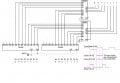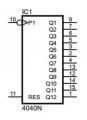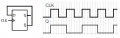#### Eshawn

Joined Oct 5, 2019
31
Hello electronics experts:

I am experimenting a circuit for adding two numbers represented by two digital counters and two cascaded 4 bits adders (74hc283). I tested separately with cascaded 74hc163 and with asynchronous 74hc4040 counters and I ended up with the same results.

I fee one of the counters (at pin 10) with a 1 khz clk signals and the second counter with 512 hz signals. All signals are well formed square signals.

I use 16hz crystal-controlled time base. The counters count during logic high of the time base that is 1/32 sec or about 31 msec. The counters are reset (at pin 11) in the second half of the logic-low of the time base.

When I verify the outputs of the first counter, my prob realizes pulses at some of the outputs and I consider them as logic high. So that the output of the first counter is binary 00011111 to represent the 1khz clk input, almost as expected. The output of the second counter is binary 00001111 to represent the 512 hz clk input also during the 1/32 sec. So far so good.I feed one of the counters with a 1 khz com signals and the second counter with 512 hz signals. All signals are well formed square signals.

I use 16hz crystal-controlled time base. The counters count during logic high of the time base that is 1/32 sec or about 31 msec. The counters are reset in the second half of the logic-low of the time base.

When I verify the outputs of the first counter, my oscilloscope realizes pulses at some of the outputs and I consider them as logic high. So that the output of the first counter is binary 00011111 to represent the 1khz clk input, almost as expected. The output of the second counter is binary 00001111 to represent the 512 hz clk input also during the 1/32 sec. So far so good because I can read the output of the counters correctly.

I feed input a[] of the cascaded adders by the outputs of the first counter and input b[] of the adders by outputs of the second counter. I expect to see the same pulsing output pins of the adders to show correct sum. My expectation is to see the correct sum as binary number 00101110 at output s[] of the 8 bits adder, the same way that I see the correct binary numbers at the pulsing pins of the two counters. But instead, I see binary 00111111 as the sum. If I change the clk input of the first counter to 2khz and I leave the clk input of the second counter as it was 512 hz, I see binary number 01111111 instead of the correct sum 01001110 as sum at outputs s[] of the adder. If I change the clk input of the first counter to 512khz like the second counter, I see the correct output of 00011110 as sum at outputs s[] of the adder.

I understand that as the counter is cycling through the addition is performed. But my thoughts are that the total propagation delay of the counters and the adders is in the order of hundreds of nanoseconds but the counters are not counting for many milliseconds before they are reset. My expectation is to see the correct sum at output s[] of the adder at their pulsing output pins while the counters are counting during the logic high of the time base? I mean, I expect to see the right output of the adders at their pulsing output pins just like I can see the right output of the counters at their pulsing output pins. Is my expectation justified while counters periodically pause counting? If so, why do I get wrong sum?

Please note that it is not about what kind of counters I am using. It is about the wrong sum no matter which counter I use.

Last edited:

#### djsfantasi

Joined Apr 11, 2010
9,131

#### Deleted member 115935

Joined Dec 31, 1969
0
I am experimenting a circuit with two digital counters and two cascaded 4 bits adders (74hc283). I tested separately with cascaded 74hc163 and with asynchronous 74hc4040 counters and I ended up with the same questions.

I clk one of the counters with a 1 khz signals and the second counter with 512 hz signals. All signals are well formed square signals.

I use 16hz crystal-controlled time base. The counters count during logic high of the time base that is 1/32 sec or about 31 msec. The counters are reset in the second half of the logic-low of the time base.

When I verify the outputs of the first counter, my prob realizes pulses at some of the outputs and I consider them as logic high. So that the output of the first counter is binary 00011111 to represent the 1khz clk input, almost as expected. The output of the second counter is binary 00001111 to represent the 512 hz clk input also during the 1/32 sec. So far so good.

My expectation is to see the correct sum as binary number 00101110 at output s[] of the 8 bits adder, the same way that I see the correct binary numbers at the outputs of the two counters. But instead, I see binary 00111111 as the sum. If I change the clk input of the first counter to 2khz and I leave the clk input of the second counter as it was 512 hz, I see binary number 01111111 instead of the correct sum 01001110 as sum at outputs s[] of the adder. If I change the clk input of the first counter to 512khz like the second counter, I see the correct output of 00011110 as sum at outputs s[] of the adder

Is my expectation to see the correct sum at output s[] of the adder justified while the counters are counting during the logic high of the time base?

If so, why do I get wrong sum?

If my expectation is wrong then how am I supposed to get the right sum while the counters are counting during the logic high of the time base?

Because I use low clk frequency, I’d rather to use the 4040 counters for simplicity.

Because I have difficulties to provide a schematic without having a right software, I described the circuit as it is. As a note, I ground the carry-in pin of the first 4 bits adder and I connect the carry-out pin of the first adder to the carry-in of the second adder.

I hope my question is clear enough to receive a helpful response.
If you have a schematic please take a picture of it , or if its on windows, try printing a PDF to post or use the "snip tool" of windows.

#### Eshawn

Joined Oct 5, 2019
31
I am experimenting a circuit with two digital counters and two cascaded 4 bits adders (74hc283). I tested separately with cascaded 74hc163 and with asynchronous 74hc4040 counters and I ended up with the same questions.

I clk one of the counters with a 1 khz signals and the second counter with 512 hz signals. All signals are well formed square signals.

I use 16hz crystal-controlled time base. The counters count during logic high of the time base that is 1/32 sec or about 31 msec. The counters are reset in the second half of the logic-low of the time base.

When I verify the outputs of the first counter, my prob realizes pulses at some of the outputs and I consider them as logic high. So that the output of the first counter is binary 00011111 to represent the 1khz clk input, almost as expected. The output of the second counter is binary 00001111 to represent the 512 hz clk input also during the 1/32 sec. So far so good.

My expectation is to see the correct sum as binary number 00101110 at output s[] of the 8 bits adder, the same way that I see the correct binary numbers at the outputs of the two counters. But instead, I see binary 00111111 as the sum. If I change the clk input of the first counter to 2khz and I leave the clk input of the second counter as it was 512 hz, I see binary number 01111111 instead of the correct sum 01001110 as sum at outputs s[] of the adder. If I change the clk input of the first counter to 512khz like the second counter, I see the correct output of 00011110 as sum at outputs s[] of the adder

Is my expectation to see the correct sum at output s[] of the adder justified while the counters are counting during the logic high of the time base?

If so, why do I get wrong sum?

If my expectation is wrong then how am I supposed to get the right sum while the counters are counting during the logic high of the time base?

Because I use low clk frequency, I’d rather to use the 4040 counters for simplicity.

Because I have difficulties to provide a schematic without having a right software, I described the circuit as it is. As a note, I ground the carry-in pin of the first 4 bits adder and I connect the carry-out pin of the first adder to the carry-in of the second adder.

I hope my question is clear enough to receive a helpful response.

#### Eshawn

Joined Oct 5, 2019
31
If you have a schematic please take a picture of it , or if its on windows, try printing a PDF to post or use the "snip tool" of windows.

#### Eshawn

Joined Oct 5, 2019
31

#### dl324

Joined Mar 30, 2015
16,135
Because I have difficulties to provide a schematic without having a right software, I described the circuit as it is. As a note, I ground the carry-in pin of the first 4 bits adder and I connect the carry-out pin of the first adder to the carry-in of the second adder.

I hope my question is clear enough to receive a helpful response.
Obviously it isn't. Even a hand drawn description would be better than trying to describe the circuit with words.
I’d rather to use the 4040 counters for simplicity.
Decoding the outputs of asynchronous counters isn't simple. The amount of logic needed to prevent glitch hazards will be usually be more costly than using synchronous counters.

•Deleted member 115935

#### Eshawn

Joined Oct 5, 2019
31
Obviously it isn't. Even a hand drawn description would be better than trying to describe the circuit with words.
Decoding the outputs of asynchronous counters isn't simple. The amount of logic needed to prevent glitch hazards will be usually be more costly than using synchronous counters.
The problem at this point is not about synchronous or asynchronous counters. I get the same wrong sum with both counters. The question is why I get the wrong sum?

#### dl324

Joined Mar 30, 2015
16,135
The problem at this point is not about synchronous or asynchronous counters. I get the same wrong sum with both counters. The question is why I get the wrong sum?
Show us a schematic so we have a better idea of what you're working with.

What is the point of using adders?

Is this school work?

•Deleted member 115935

#### Eshawn

Joined Oct 5, 2019
31
Show us a schematic so we have a better idea of what you're working with.

What is the point of using adders?

Is this school work?
I just uploaded a schematic. And no it is not a school work.

#### Eshawn

Joined Oct 5, 2019
31
Thanks for trying. But I don’t think you can answer my question if you don’t know the counter. I need an expert who is familiar with these counters and digital addition to reply to me..

#### dl324

Joined Mar 30, 2015
16,135
Thanks for trying. But I don’t think you can answer my question if you don’t know the counter. I need an expert who is familiar with these counters and digital addition to reply to me..
The problem isn't my being able to understand how the counter works; it's your inability to draw a schematic that's intelligible.

I asked in the inputs labeled 10 and 11 were pin numbers. We don't put pin numbers inside of a logic symbol. This is a more reasonable symbol for HC4040:#### Eshawn

Joined Oct 5, 2019
31
I was hoping for someone to provide a good response for my question. Instead this thread is now crowded by where to write PIN numbers. What else could it be. If you know the 4040 counters then you should know that besides its outputs it has one pin 10 for cla input and one pin 11 for reset. Then it is not obvious to you from the obvious that the purpose is digital addition and you want to answer my question.
I am not sure if anyone can follow this crowded thread now.

#### MrChips

Joined Oct 2, 2009
29,845
You cannot feed two counters with two different clocks and expect to see any meaningful sum.
If the two clocks are in phase, yes maybe you can.
If one clock is 1kHz and the second clock is 500Hz derived from the first clock then you can observe meaningful results. You would have to examine the output using the faster of the two clocks, i.e. 1kHz clock.

#### Eshawn

Joined Oct 5, 2019
31
You cannot feed two counters with two different clocks and expect to see any meaningful sum.
If the two clocks are in phase, yes maybe you can.
If one clock is 1kHz and the second clock is 500Hz derived from the first clock then you can observe meaningful results. You would have to examine the output using the faster of the two clocks, i.e. 1kHz clock.

Would it be possible for you to answer me the following Please?

1- what do you exactly mean by examining the output using the faster of the two clocks? How this should be done?

2- what is the reason that we cannot feed two counters with two different clocks and expect to see any meaningful sum? I thought, because the counters are at rest for about 15 ms in the first half of the low-logic time base before the counters are reset, there is enough time for all propagation delays of the counters and adders to settle down while the counters are not counting in order to get a final meaningful sum. Is that a wrong thought?

#### MrChips

Joined Oct 2, 2009
29,845
You have to examine the output when both clocks have stopped, i.e. there is no clock transition present.
This is not possible with a 1kHz clock and a 512Hz clock because they are not synchronized.

If you have 500Hz derived from a 1kHz clock the waveforms of the two clocks would look like this.Note that the two waveforms are synchronized.

If you were to use either edge of the Q signal then you could run into glitches because the counter with the faster clock is still in transition. If you use the falling edge of the faster CLK then the counter with the slower clock Q is not being clocked and is stable.

#### Eshawn

Joined Oct 5, 2019
31
Thanks again.

You wrote that I have to examine the output when both clocks have stopped, and this is not possible with a 1kHz clock and a 512Hz clock because they are not synchronized. Forgive me for not understanding what you mean. As you see from my schematic, although the two clock signals are not synchronized they are stopped while time base is logic low because the clocks are gated.

For this reason I cannot understand why you think that both clocks cannot be stopped. Did I misunderstand something? Can you please clarify that why the gated clocks which are stopped for few milliseconds cannot let the counters and adders to produce a meaningful sum?

#### MrChips

Joined Oct 2, 2009
29,845
Why are you doing this?
What are you attempting to do?

#### Deleted member 115935

Joined Dec 31, 1969
0
Not certain, but assuming counter outputs a,b,c,d are the lower 4 bits of the counter, could it be that to me it looks like your feeding the Most Significant bits of the counters into the least significant bits of the adders ?

As for all the points about clock s, and needing to be synchronous to each other etc,
they all also need to be addressed.